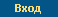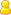Логин: Пароль: Забыли пароль?русский | englishВойти | Регистрация | Помощь

# Семинар Добрушинской математической лаборатории ИППИ РАН

Семинар Добрушинской математической лаборатории ИППИ РАН

8 декабря, вторник, 16:00 пройдет в Zoom по обычному адресу (см. ниже).

А. Магазинов: On fluctuations of random surfaces

Abstract:
Random surfaces in statistical physics are commonly modeled by a
real-valued function phi on the vertex set of a lattice-like graph,
which is chosen at random while the underlying distribution penalizes
large gradients of the function between adjacent vertices.
Precisely, given an even function V, termed the potential, the
energy H(phi) is computed as the sum of V over the nearest-neighbor
gradients of phi, and the probability density of phi is set
proportional to exp(-H(phi)). The most-studied case is when V is
quadratic, resulting in the so-called discrete Gaussian free field.
For what follows, we set the graph to be an even torus of size 2L an
dimension 3 or higher, and, fixing a vertex v_0, apply a simple
boundary condition phi(v_0) = 0. An application of the Brascamp-Lieb
inequality yields that when the potential is uniformly convex
the variance Var(phi(v)) is uniformly bounded from above,
independently of the vertex v and the size 2L of the torus.
We show that the same conclusion still holds with slightly relaxed
assumptions on V, namely, when V is convex and the inequality V""(x) > 0
holds for Lebesgue-a.e. value of x. This is a joint work with Ron Peled.

Ссылка для подключения:

https://zoom.us/j/93175142429?pwd=VDViRHNOSlZSVUM5ZU03SGZyZy8xQT09

Id: 931-7514-2429 passw=057376

Пожалуйста регистрируйте участие в Zoom по вашему имени и фамилии,
а не псевдонимом. Переименовать себя можно прямо во время сессии.

 03.12.2020 | Комеч Сергей Александрович

© Федеральное государственное бюджетное учреждение науки
Институт проблем передачи информации им. А.А. Харкевича Российской академии наук, 2021
Об институте  |  Контакты  |  Старая версия сайта